Physics - Classical Mechanics - Tensile Stress and Strain[Image 1]

Introduction

Hey it's a me again @drifter1! Today we continue with Physics and more specifically the branch "Classical Mechanics" to continue with the chapter of Equilibrium and Elasticity. In this article we will get into Tensile Stress and Strain. So, without further ado, let's dive straight into it!

Tensile Stress

Tensile stress is defined as the resistance an object has to forces tending to break it. It is equal to the force per unit area that a material can endure without tearing apart. The maximum stress that the material can stand is called the ultimate tensile stress or breaking stress.

Stress is defined by the following formula: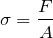• σ = tensile stress measured in N/m2 or pascals (Pa)
• F = force in newtons (N)
• A = cross-sectional area in m2
Lots of mechanical properties of materials can be determined from a tensile test. When a tensile force acts on a material, the following tensile properties can be calculated:
• Elastic modulus
• Ultimate tensile stress (UTS)
• Modulus of resilience
• Fracture stress

Tensile Strain

The ratio of extension to original length is called strain and has no units. Mathematically, we can write it as following: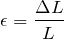• ε = tensile strain with no units
• ΔL = extension measured in metres (m)
• L = original length measured in metres (m)

Young's Modulus

Young's modulus of elasticity is defined as "The mechanical property of a material to withstand the compression or elongation with respect to its length". It is denoted as E or Y. Using Hooke's Law of elasticity, Young's modulus is defined as: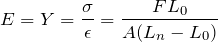• σ = tensile stress
• ε = tensile strain
• F = tensile force
• A = cross-sectional area
• L0 = original length
• Ln = new length

Poisson's Ratio

Stretching a material in one diretion it tends to get thinner, whilst compressing it tends to make it thicker in the lateral direction. Poisson's ratio is expressed as: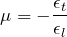• μ = Poisson's ratio
• εt = transverse strain
• εl = longitudinal or axial strain
Longitudinal (or axial) strain can be expressed as: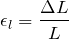• εt = longitudinal or axial strain
• ΔL = change in length
• L = initial length
Contraction (or transverse, lateral or radial) strain can be expressed as: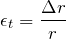• εl = transverse, lateral or radial strain
• Δr = change in radius

RESOURCES:

Images

1.Final words | Next up

And this is actually it for today's post!

Next time we will continue with Volumetric Tension and Strain...

See ya!Keep on drifting!

H2
H3
H4
3 columns
2 columns
1 column